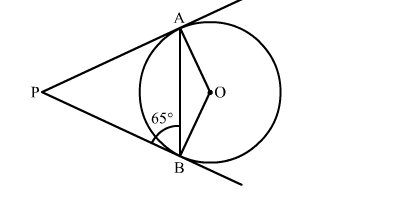# In the given figure, PA and PB are two tangents from an external point P to a circle with centre O.`
Question:

In the given figure, PA and PB are two tangents from an external point P to a circle with centre O. If ∠PBA = 65 , find ∠OAB and ∠APBSolution:

We know that tangents drawn from the external point are congruent.
∴ PA = PB
Now, In isoceles triangle APB
∠APB + ∠PBA + ∠PAB = 180            [Angle sum property of a triangle]
⇒ ∠APB + 65 + 65 = 180                          [∵∠PBA = ∠PAB = 65 ]
⇒ ∠APB = 50
We know that the radius and tangent are perperpendular at their point of contact
∴∠OBP = ∠OAP = 90